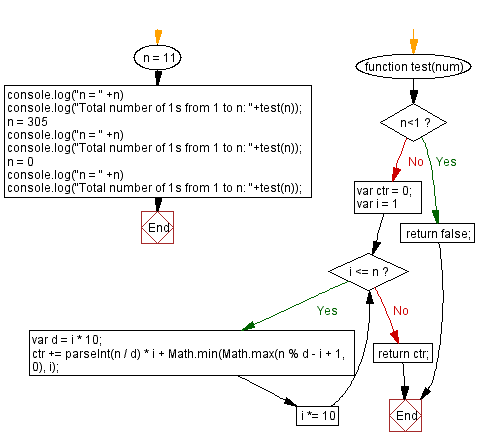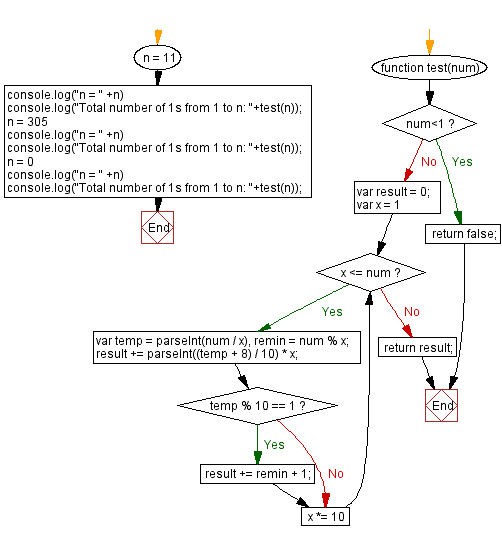# JavaScript: Count total number of 1s from 1 to N

## JavaScript Math: Exercise-102 with Solution

Write a JavaScript program to count the number of times the digit 1 appears in 1 to a given number.

Example : 11, 1 appears 4 time between 1 to 11 [1->1, 10->1 ,11-.2]

Test Data:
(11) -> 4
(305) -> 161
(0) -> false

Sample Solution:

HTML Code:

``````<!DOCTYPE html>
<html>
<meta charset="utf-8">
<title>JavaScript program to Count total number of 1s from 1 to N</title>
<body>

</body>
</html>
```
```

Solution-1

JavaScript Code:

``````function test(num) {
if (n<1)
return false;
var ctr = 0;
for (var i = 1; i <= n; i *= 10) {
var d = i * 10;
ctr += parseInt(n / d) * i +
Math.min(Math.max(n % d - i + 1, 0), i);
}
return ctr;
}
n = 11
console.log("n = " +n)
console.log("Total number of 1s from 1 to n: "+test(n));
n = 305
console.log("n = " +n)
console.log("Total number of 1s from 1 to n: "+test(n));
n = 0
console.log("n = " +n)
console.log("Total number of 1s from 1 to n: "+test(n));
```
```

Sample Output:

```n = 11
Total number of 1s from 1 to n: 4
n = 305
Total number of 1s from 1 to n: 161
n = 0
Total number of 1s from 1 to n: false
```

Flowchart:Live Demo:

See the Pen javascript-math-exercise-102 by w3resource (@w3resource) on CodePen.

Solution-2

JavaScript Code:

``````function test(num) {
if (num<1)
return false;
var result = 0;
for (var x = 1; x <= num; x *= 10) {
var temp = parseInt(num / x), remin = num % x;
result += parseInt((temp + 8) / 10) * x;
if (temp % 10 == 1)
result += remin + 1;
}
return result;
}
n = 11
console.log("n = " +n)
console.log("Total number of 1s from 1 to n: "+test(n));
n = 305
console.log("n = " +n)
console.log("Total number of 1s from 1 to n: "+test(n));
n = 0
console.log("n = " +n)
console.log("Total number of 1s from 1 to n: "+test(n));
```
```

Sample Output:

```n = 11
Total number of 1s from 1 to n: 4
n = 305
Total number of 1s from 1 to n: 161
n = 0
Total number of 1s from 1 to n: false
```

Flowchart:Live Demo:

See the Pen javascript-math-exercise-102-1 by w3resource (@w3resource) on CodePen.

Improve this sample solution and post your code through Disqus

What is the difficulty level of this exercise?

Test your Programming skills with w3resource's quiz.

﻿